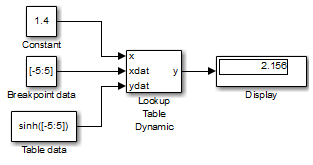## 逼近函数值的方法

### 内插方法

• `Flat`” - 禁用内插，并使用名为 “`Use Input Below`” 的舍入操作。有关详细信息，请参阅 舍入方法

• `Nearest`” - 禁用内插，并返回与最接近输入的断点对应的表值。如果输入与两个相邻断点之间的距离相等，则选择索引值较大的断点。

• `Linear point-slope`” - 在相邻断点之间拟合一条线，并返回该线条上与输入对应的点。以下是线性点-斜率的公式，其中 x 是输入数据，y 是输出表数据（xi,yi 是表数据的坐标），f 是分式。有关 xi,yi 的详细信息，请参阅 关于查找表模块

`$f=\frac{x-{x}_{i}}{{x}_{i+1}-{x}_{i}}$`
`$y={y}_{i}+f\left({y}_{i+1}-{y}_{i}\right)$`
• `Cubic spline`” - 在相邻断点之间拟合一条三次样条曲线，并返回该样条曲线上与输入对应的点。

• `Linear Lagrange`” - 使用一阶拉格朗日插值在相邻断点之间拟合一条线，并返回该线条上与输入对应的点。以下是线性拉格朗日方程，其中 x 是输入数据，y 是输出表数据，f 是分式。f 限制在 0 到小于 1 的范围内 (`[0,1)`)。有关 x 和 y 的详细信息，请参阅关于查找表模块

`$f=\frac{x-{x}_{i}}{{x}_{i+1}-{x}_{i}}$`
`$y=\left(1-f\right){y}_{i}+f{y}_{i+1}$`

如果外插方法是 “`Linear`”，则基于所选择的线性插值方法计算外插值。例如，如果插值方法是线性拉格朗日，则外插方法继承线性拉格朗日方程以计算外插值。

• `Akima spline`” - 在相邻断点之间拟合一条 Akima 样条曲线，并返回该样条曲线上与输入对应的点。插值方法仅适用于 “`Akima spline`” 外插方法。修正 Akima 三次 Hermite 插值方法具有以下属性：

• 它比三次样条产生的波动更少。

• 对于实时应用，它比三次样条更高效。

• 与三次样条不同，它不会产生过冲。

• 与三次样条不同，它支持非标量信号。

Lookup Table Dynamic 模块不允许您选择内插方法。模块参数对话框中的 Lookup Method 字段中的 “`Interpolation-Extrapolation`” 选项会执行线性插值。

### 外插方法

• `Clip`” - 禁用外插，并返回与断点数据集范围的端点对应的表数据。此方法对超出范围的值无任何保证。

• `Linear`” - 如果插值方法为 “`Linear`”，此外插方法将在第一对断点间或最后一对断点间拟合一条线，具体取决于输入是小于第一个断点还是大于最后一个断点。如果插值方法为 “`Cubic spline`” 或 “`Akima spline`”，此外插方法将使用插值在第一个或最后一个断点处的斜率来拟合一个线性曲面，具体取决于输入是小于第一个断点还是大于最后一个断点。外插方法将返回生成的线性曲面上与输入对应的点。

如果外插方法是 “`Linear`”，则基于所选择的线性插值方法计算外插值。例如，如果插值方法是线性拉格朗日，则外插方法继承线性拉格朗日方程以计算外插值。

• `Cubic spline`” - 对第一对或最后一对断点拟合一条三次样条曲线，具体取决于输入是小于第一个断点还是大于最后一个断点。此方法将返回该样条曲线上与输入对应的点。

• `Akima spline`” - 分别对第一对或最后一对断点拟合一条 Akima 样条曲线，具体取决于输入是小于第一个断点还是大于最后一个断点。此方法将返回该样条曲线上与输入对应的点。

Lookup Table Dynamic 模块不允许您选择外插方法。模块参数对话框中的 Lookup Method 字段中的 “`Interpolation-Extrapolation`” 选项会执行线性外插。

### 舍入方法

• `Use Input Nearest`” - 返回与最接近的输入值对应的输出值。

• `Use Input Below`” - 返回与小于输入值的紧邻断点值对应的输出值。如果输入值下面没有任何断点值，则返回最接近输入值的断点值。

• `Use Input Above`” - 返回与大于输入值的紧邻断点值对应的输出值。如果输入值上面没有任何断点值，则返回最接近输入值的断点值。

### 各查找方法的输出示例Lookup Method输入输出注释

```Interpolation- Extrapolation```

`1.4`

`2.156`

`5.2`

`83.59`

```Interpolation- Use End Values```

`1.4`

`2.156`

`5.2`

`74.2`

`Use Input Above`

`1.4`

`3.627`

`5.2`

`74.2`

`Use Input Below`

`1.4`

`1.175`

`-5.2`

`-74.2`

`Use Input Nearest`

`1.4`

`1.175`